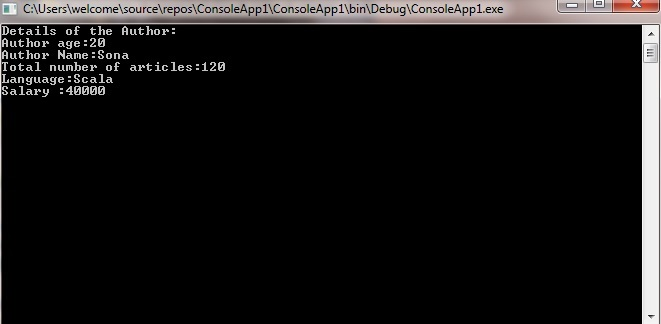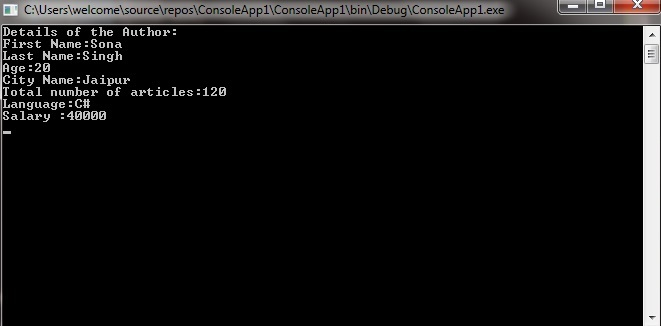# C# | Deconstructor with Classes

In Classes, deconstructor is used in the form of methods to access the class variables outside the class by assigning them into new variables. It is achieved by using out parameters because using out parameters you can have multiple overloads for different values. Also, you are allowed to use multiple deconstructor methods in the same program with the same number of out parameters or the same number and type of out parameters in a different order, but be careful with such type of multiple deconstructor methods because they cause a lot of confusion.

Syntax:

```// Creating a deconstructor method
public void Deconstruct( out T var1, out T var2, ..., out T varN)
{
// Code..
}
// Deconstructor assignment
(T var1, ..., T varN) = obj

var(_, var2, _, var4) = obj
```

Here T is the types of the variables. Let us understand the concept of deconstructors with the help of the given examples:

Example 1:

 `// C# program to illustrate the concept ` `// of deconstruction with class ` `using` `System; ` `using` `System.Collections.Generic; ` `using` `System.Linq; ` `using` `System.Text; ` `using` `System.Threading.Tasks; ` ` `  `namespace` `ConsoleApp1 ` `{ ` `    ``// Geeks class ` `    ``public` `class` `Geeks ` `    ``{ ` `        ``// Creating variables ` `        ``string` `Aname; ` `        ``int` `age; ` `        ``int` `Tarticals; ` `        ``string` `language; ` `        ``double` `salary; ` ` `  `        ``// Method ` `        ``public` `Geeks( ``string` `_AuthorName, ``int` `_Age, ``int` `_TotalArticals,  ` `                                      ``string` `_Language, ``double` `_Salary) ` `        ``{ ` `            ``age = _Age; ` `            ``Aname = _AuthorName; ` `            ``Tarticals = _TotalArticals; ` `            ``language = _Language; ` `            ``salary = _Salary; ` `        ``} ` ` `  `        ``// Deconstructor  ` `        ``public` `void` `Deconstruct( ``out` `string` `_AuthorName, ``out` `int` `_Age, ` `                         ``out` `int` `_TotalArticals, ``out` `string` `_Language,  ` `                                                   ``out` `double` `_Salary) ` `        ``{ ` `             `  `            ``_AuthorName = Aname; ` `            ``_Age = age; ` `            ``_TotalArticals = Tarticals; ` `            ``_Language = language; ` `            ``_Salary = salary; ` `        ``} ` `    ``} ` `     `  `public` `class` `GFG ` `{ ` `    ``// Main method ` `    ``static` `public` `void` `Main() ` `    ``{ ` `        ``// Creating object of Geeks class ` `        ``Geeks obj = ``new` `Geeks( ``"Sona"``, 20, 120, ``"Scala"``, 40000.0); ` ` `  `        ``// Deconstruct the instance of  ` `        ``// the Geeks class named as obj ` `        ``(``string` `AuthorName, ``int` `Age, ``int` `TotalArticals,  ` `                 ``string` `Language, ``double` `Salary) = obj; ` ` `  `        ``// Displayin the values ` `        ``Console.WriteLine(``"Details of the Author:"``); ` `        ``Console.WriteLine(``"Author age:{0}"``, Age); ` `        ``Console.WriteLine(``"Author Name:{0}"``, AuthorName); ` `        ``Console.WriteLine(``"Total number of articles:{0}"``, TotalArticals); ` `        ``Console.WriteLine(``"Language:{0}"``, Language); ` `        ``Console.WriteLine(``"Salary :{0}"``, Salary); ` `        ``Console.ReadLine(); ` `    ``} ` `} ` ` `  `} `

Output:Example 2:

 `// C# program to illustrate the concept of ` `// multiple deconstruction with class ` `using` `System; ` `using` `System.Collections.Generic; ` `using` `System.Linq; ` `using` `System.Text; ` `using` `System.Threading.Tasks; ` `  `  `namespace` `ConsoleApp1 ` `{ ` `    ``// Geeks class ` `    ``public` `class` `Geeks ` `    ``{ ` `        ``// Creating variables ` `        ``string` `Afname; ` `        ``string` `Alname; ` `        ``string` `city; ` `        ``int` `age; ` `        ``int` `Tarticals; ` `        ``string` `language; ` `        ``double` `salary; ` `  `  `        ``// Method ` `        ``public` `Geeks( ``string` `_AuthorFirstName, ``string` `_AuthorLastName, ` `                          ``string` `_City,  ``int` `_Age, ``int` `_TotalArticals, ` `                                     ``string` `_Language, ``double` `_Salary) ` `        ``{ ` `            ``age = _Age; ` `            ``Afname = _AuthorFirstName; ` `            ``Alname = _AuthorLastName; ` `            ``city = _City; ` `            ``Tarticals = _TotalArticals; ` `            ``language = _Language; ` `            ``salary = _Salary; ` `        ``} ` `  `  `        ``// Deconstructor 1 ` `        ``public` `void` `Deconstruct(``out` `string` `_AuthorFirstName,  ` `               ``out` `string` `_AuthorLastName, ``out` `string` `_City) ` `        ``{ ` `  `  `            ``_AuthorFirstName = Afname; ` `            ``_AuthorLastName = Alname; ` `            ``_City = city; ` `        ``} ` `  `  `        ``// Deconstructor 2 ` `        ``public` `void` `Deconstruct(  ``out` `int` `_Age, ``out` `int` `_TotalArticals,  ` `                              ``out` `string` `_Language, ``out` `double` `_Salary) ` `        ``{ ` `            ``_Age = age; ` `            ``_TotalArticals = Tarticals; ` `            ``_Language = language; ` `            ``_Salary = salary; ` `        ``} ` `    ``} ` `     `  `     `  `public` `class` `GFG ` `{ ` `    ``// Main method ` `    ``static` `public` `void` `Main() ` `    ``{ ` `        ``// Creating object of Geeks class ` `        ``Geeks obj = ``new` `Geeks( ``"Sona"``, ``"Singh"``,  ` `             ``"Jaipur"``, 20, 120, ``"C#"``, 40000.0); ` ` `  `        ``// Deconstruct the instance of ` `        ``// the Geeks class named as obj ` `        ``( ``int` `Age, ``int` `TotalArticals, ``string` `Language, ``double` `Salary) = obj; ` `        ``(``string` `AuthorFirstName, ``string` `AuthorLastName, ``string` `City) = obj; ` ` `  `        ``// Displaying the values ` `        ``Console.WriteLine(``"Details of the Author:"``); ` `        ``Console.WriteLine(``"First Name:{0}"``, AuthorFirstName); ` `        ``Console.WriteLine(``"Last Name:{0}"``, AuthorLastName); ` `        ``Console.WriteLine(``"Age:{0}"``, Age); ` `        ``Console.WriteLine(``"City Name:{0}"``, City); ` `        ``Console.WriteLine(``"Total number of articles:{0}"``, TotalArticals); ` `        ``Console.WriteLine(``"Language:{0}"``, Language); ` `        ``Console.WriteLine(``"Salary :{0}"``, Salary); ` `        ``Console.ReadLine(); ` `    ``} ` `} ` `  `  `} `

Output:My Personal Notes arrow_drop_upCheck out this Author's contributed articles.

If you like GeeksforGeeks and would like to contribute, you can also write an article using contribute.geeksforgeeks.org or mail your article to contribute@geeksforgeeks.org. See your article appearing on the GeeksforGeeks main page and help other Geeks.

Please Improve this article if you find anything incorrect by clicking on the "Improve Article" button below.

Article Tags :

Be the First to upvote.

Please write to us at contribute@geeksforgeeks.org to report any issue with the above content.